×

Search anything:

# Call by Value vs Call by Reference in C++

#### Software Engineering C++Get this book -> Problems on Array: For Interviews and Competitive Programming

'Call' refers to calling a function. Both of these (Call by Value and Call by Reference) are methods of function invocation. The difference, lies in formal parameters. We have explored the difference in depth in C++.

## Parameters

The term parameter refers to any declaration within the parentheses. This maybe observered during function invokation or declaration.

A function is a set of statements that may take input(s) to perform predetermined tasks and produce desired output. It receives input in the form of parameters.

Example:

``````int sum(int a,int b, int c)
{
add = a + b + c;
}
``````

Above is a function to produce sum of 2 or 3 numbers, wherein
Input variables- a, b, c
Their scope- Function body
wherein scope of a variable is defined as the extent of the program code within which the variable is accessed, declared & worked with.

Here you can only access these parameters a, b, c in the function body and not in any other function or the main function.

'a, b, c' are formal parameters!

Formal parameters are the variables defined by the function which receive values when the function is called.

This function maybe invoked by using integer variables x, y, z as follows

``````int s = sum(x,y,z);
``````

'x, y, z' are known as actual parameters

Actual Parameters are values that are passed to a function when invoked.

## Call by Value

Call by value is a method of function invokation when the values of formal parameters get copied to the actual parameters.

Example-

``````#include<iostream>
using namespace std;
int sum(int a,int b, int c)
{
add = a + b + c;
}
int main()
{
int x = 5, y = 3, z = 2;
int s = sum(x,y,z);
cout<<s;
return 0;
}
``````

Output-

``````10
``````

The values of x, y, z get copied to a, b, c respectively.

Both sets of parameters function independently of each other and have different scope.
Hence any changes in the formal parameters will not reflect back to the actual parameters.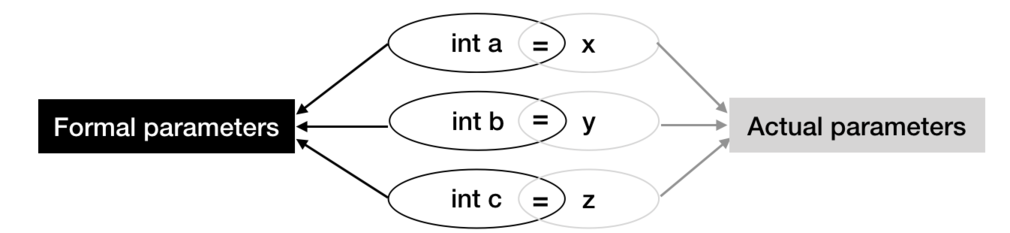Note: As evident by above configuration formal parameters must contain the datatype, whereas the actual parameters are only might as well only contain values.

Example

``````int s = sum(3,3*2,1);
``````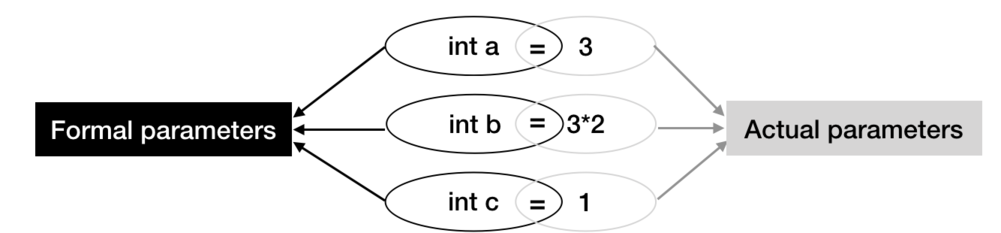## Call by Reference

Here,

References are an alternative way to refer a variable.
Syntax for declaration:

``````type &reference_name = variable name
``````

Example:

``````int &b = a;
``````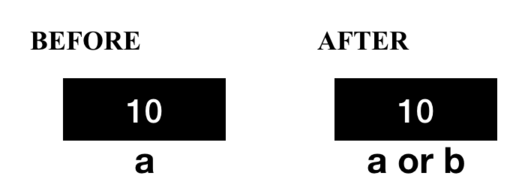So ofcourse, any changes made to 'b' will also be made to 'a', b is known to be alias name of a.

In call by reference method formal parameters are references of actual parameters.

The sum function shall be altered as follows

``````int sum(int &a,int &b, int &c)
{
add = a + b + c;
}
``````

This shall be translated to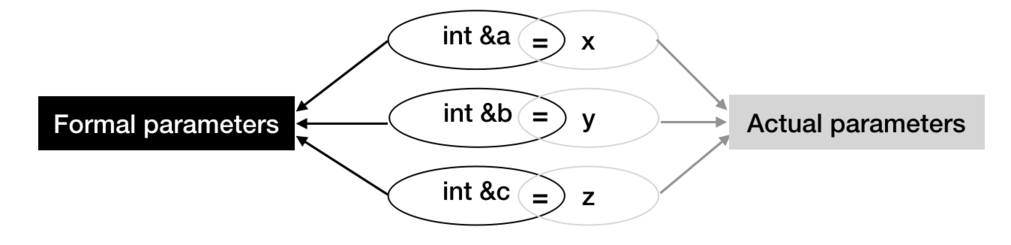a, b, c are references to x, y, z and no new variables are declared.

Hence any changes in the formal parameters will reflect back to the actual parameters.

Take care: Order, number and type of actual arguments in the function call must match the formal arguments of the function.

### Illustrating as follows

Lets define functions for swapping variables.

``````#include<iostream>
using namespace std;
void swap_val(int a, int b)
{
int temp;
temp=a;
a=b;
b=temp;
cout<<"After swapping by value the formal parameters are as follows"<<endl;
cout<<" a = "<<a<<endl<<" b = "<<b<<endl;
}
void swap_ref(int &a, int &b)
{
int temp;
temp=a;
a=b;
b=temp;
cout<<"After swapping by reference the formal parameters are as follows"<<endl;
cout<<" a = "<<a<<endl<<" b = "<<b<<endl;
}
int main()
{
int x = 5, y= 6;
cout<<"My actual parameters are \n x = "<<x<<endl<<" y = "<<y<<endl;
swap_val(x,y);
cout<<"My actual parameters are \n x = "<<x<<endl<<" y = "<<y<<endl;
swap_ref(x,y);
cout<<"My actual parameters are \n x = "<<x<<endl<<" y = "<<y<<endl;
return 0;
}
``````

OUTPUT:

``````My actual parameters are
x = 5
y = 6
After swapping by value the formal parameters are as follows
a = 6
b = 5
My actual parameters are
x = 5
y = 6
After swapping by reference the formal parameters are as follows
a = 6
b = 5
My actual parameters are
x = 6
y = 5
``````

You may observe the actual parameters remain unchanged in case of Call by value whereas they get swapped in case of Call by Reference.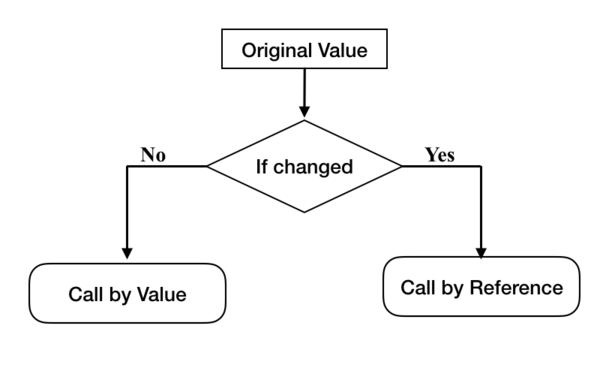This is evident as in call by value, a new set of variables are generated in call by value which are independent of the actual parameters whereas in call by reference formal parameters are just alias names of actual parameters and essentially refer to the same memory location.

### Differences between the two methods

All differences are direct consequences of the primary difference that we're passing values in 'Call by Value' and passing references or address of variables in 'Call by Reference'.

• Call by Value- We cannot alter values of actual variables through function calls
Call by Reference- The ability to change the value of argument is rendered.

• Call by Value- Actual value is preserved. It cannot be accidentally modified by the function as well.
Call by Reference- Actual values undergo the same fate as the formal parameters do.

• Call by Value uses extra space for formal parameters and making Call by Reference more memory efficient.

• Since no copies are being made in Call by Reference, it is faster than Call by Value.

## Question

#### Guess the Output

``````#include<iostream>
using namespace std;
void f1(int a , int &b)
{
int temp;
temp = a;
a = b;
b = temp;
}
int main()
{
int x = 5, y = 6;
f1(x,y);
cout<<"x = "<<x<<" y = "<<y;
return 0;
}
``````
x = 5 y = 5
x = 6 y = 6
x = 6 y = 5
x = 5 y = 6
The first parameter is passed by value and the second parameter is passed by reference, so the changes in first formal parameter is not reflected in the actual parameter, so x remains to be 5. In function definition, the second parameter gets the value of a, i.e. 5. Since that is passed by reference it shall contain the value of y, and so it is also equal to 5.

By this article at OpenGenus, you must be clear with concept of Call by Value and Call by Reference. Enjoy.#### Priyanshi Sharma

Priyanshi Sharma has been an Intern at Google in 2021 | Computer Science student at National Institute of Technology, Raipur | 2020 Intern at OpenGenus | Author of "Problems in AI" |

Call by Value vs Call by Reference in C++